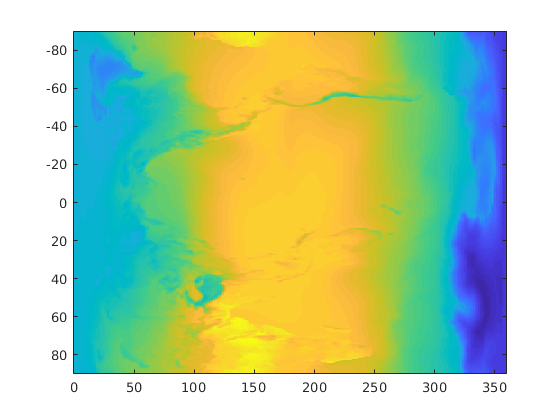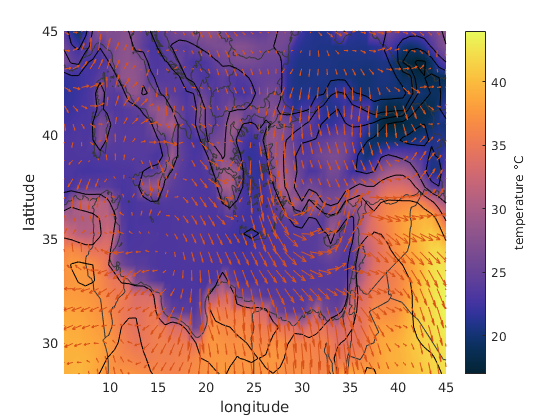# Working with NetCDF data

Back to Climate Data Tools Contents

NetCDF is one of the most common data formats in climate science, and it's a pretty easy format to work with in Matlab, but it helps to know a few tricks. Here's a step-by-step guide to reading and analyzing NetCDF data into Matlab.

## Contents

The example data used in this tutorial is provided as part of CDT, so you don't need to download anything. But acquiring data is often the first step, so for posterity's sake here's how I downloaded the sample data:

1. Login to the ECMWF website and found ERA-Interim Synoptic Monthly Means.
2. Select times 00:00:00, 12 step, and the fields 2 m temperature, 10 m U and V wind components, surface pressure, and total precipitation.
3. Select NetCDF data format and download at the default 0.75x0.75 degree resolution.
4. Wait about 15 minutes for the data to be ready and download it.
5. The default filenames for data downloaded from ECMWF are often long and cryptic, so for this tutorial I renamed it ERA_Interim_2017.nc.

After downloading the data, put it either in your current folder or somewhere else Matlab can find it. Consider creating a folder just for data, and add that folder to Matlab's search path via addpath.

## Check the contents of the NetCDF file

The first step in loading NetCDF data is figuring out what variable names are in the file. To do that, use ncdisp like this:

```ncdisp 'ERA_Interim_2017.nc'
```
```Source:
Format:
64bit
Global Attributes:
Conventions = 'CF-1.6'
history     = '2018-12-06 18:53:30 GMT by grib_to_netcdf-2.9.2: grib_to_netcdf /data/data01/scratch/78/bb/_mars-atls17-98f536083ae965b31b0d04811be6f4c6-L3jQQq.grib -o /data/data03/scratch/77/57/_grib2netcdf-atls19-98f536083ae965b31b0d04811be6f4c6-5zbphu.nc -utime'
Dimensions:
longitude = 480
latitude  = 241
time      = 12    (UNLIMITED)
Variables:
longitude
Size:       480x1
Dimensions: longitude
Datatype:   single
Attributes:
units     = 'degrees_east'
long_name = 'longitude'
latitude
Size:       241x1
Dimensions: latitude
Datatype:   single
Attributes:
units     = 'degrees_north'
long_name = 'latitude'
time
Size:       12x1
Dimensions: time
Datatype:   int32
Attributes:
units     = 'hours since 1900-01-01 00:00:00.0'
long_name = 'time'
calendar  = 'gregorian'
sp
Size:       480x241x12
Dimensions: longitude,latitude,time
Datatype:   int16
Attributes:
scale_factor  = 0.79238
_FillValue    = -32767
missing_value = -32767
units         = 'Pa'
long_name     = 'Surface pressure'
standard_name = 'surface_air_pressure'
u10
Size:       480x241x12
Dimensions: longitude,latitude,time
Datatype:   int16
Attributes:
scale_factor  = 0.00041182
_FillValue    = -32767
missing_value = -32767
units         = 'm s**-1'
long_name     = '10 metre U wind component'
v10
Size:       480x241x12
Dimensions: longitude,latitude,time
Datatype:   int16
Attributes:
scale_factor  = 0.00041589
_FillValue    = -32767
missing_value = -32767
units         = 'm s**-1'
long_name     = '10 metre V wind component'
t2m
Size:       480x241x12
Dimensions: longitude,latitude,time
Datatype:   int16
Attributes:
scale_factor  = 0.0017972
_FillValue    = -32767
missing_value = -32767
units         = 'K'
long_name     = '2 metre temperature'
tp
Size:       480x241x12
Dimensions: longitude,latitude,time
Datatype:   int16
Attributes:
scale_factor  = 6.832e-07
_FillValue    = -32767
missing_value = -32767
units         = 'm'
long_name     = 'Total precipitation'
```

The output of ncdisp tells us everything we need to know. Most imporantly, it tells us the names of the variable in the NetCDF file, and also includes notes about the units of each variable.

Notice the Size of each variable. That's usually a first clue into how the data matrices are organized. The longitude variable is 480x1, latitude is 241x1, and time is 12x1. And it's no coincidence that the other variables are 480x241x12: these dimensions correspond to longitude, latitude, and time.

Some variables include a scale_factor, add_offset, and _FillValue. We don't need to pay attention to those values because Matlab's ncread function automatically does the scaling and offsetting, but in case you're curious, those values are what the algorithm uses to pack (and unpack) the data efficiently into NetCDF format.

## Read the data

Now that we know the names of the variables in ERA_Interim_2017.nc, we can load the ones that interest us. Let's look at the air temperature taken at 2 m ('t2m'), and get its corresponding geographic coordinates:

```lat = ncread('ERA_Interim_2017.nc','latitude');
```

## Read the time array

The easiest way to read time arrays from NetCDF files is to use the CDT function ncdateread. It works just like ncread, but automatically converts time arrays to datetime format (read more about time formats here). Here's how to load time with ncdateread:

```t = ncdateread('ERA_Interim_2017.nc','time');
```

## Plot the data:

This tutorial describes several tricks for making fancy maps, but right now let's just use the imagesc function to check out the grid orientation. That means we'll let lon be the x variable and let lat be the y variable.

For the T variable we know the third dimension corresponds to time, so take the mean of T along the third dimension to get a map of mean temperature for 2017:

```% Calculate mean temperature of 2017:
Tm = mean(T,3);

% Plot mean temperature:
imagesc(lon,lat,Tm)
```## Rotate and flip the grid

The grid in the plot above is rotated! That's not uncommon when importing NetCDF and other data formats, which often use the first dimension as the "x" dimension and the second dimension as the "y" dimension. One way to deal with this is to rotate the matrix with rot90 and then flip it vertically with flipud:

```% Rotate and flip T:
T = flipud(rot90(T));

% Recalculate Tm using the rotated, flipped data matrix:
Tm = mean(T,3);

% Re-plot the mean temperature:
imagesc(lon,lat,Tm);
```Now it's rotated, but it's still upside down. So why'd we flip it? Because of an idiosyncrasy of imagesc, which needs to be followed with the axis xy command whenever you specify x,y coordinates:

```axis xy
```## Getting coordinates for each grid cell

So far we've been using lat and lon as vectors, with the simple assumption that each value in lat corresponds to a row of T, and each value in lon corresponds to a column of T. But sometimes you need lat and lon to be full grids. For that, use meshgrid.

```[Lon,Lat] = meshgrid(lon,lat);
```

Note the order of lat and lon when using meshgrid. If we had not rotated the dimensions of the grid earlier, we would use [Lat,Lon] = meshgrid(lat,lon) instead.

With full grids for coordinates instead of 1D arrays, use pcolor instead of imagesc. Also, pcolor is picky and wants the coordinates to be in double precision, so convert as you see here:

```pcolor(double(Lon),double(Lat),Tm)
shading interp % eliminates black lines between grid cells
cmocean thermal % cold-to-hot colormap
xlabel longitude
ylabel latitude
```## Recenter the grid

You may have also noticed that the longitudes go from 0 to 360, which puts the Pacific Ocean in the middle of the map. Use recenter to shift the grid such that the prime meridian is in the middle.

```[Lat,Lon,T,Tm] = recenter(Lat,Lon,T,Tm);

pcolor(double(Lon),double(Lat),Tm)
shading interp % eliminates black lines between grid cells
hold on
borders('countries','color',rgb('dark gray'))
cmocean thermal % cold-to-hot colormap
xlabel longitude
ylabel latitude
```## (Advanced) Save memory: Read only the data you need

Sometimes NetCDF datasets are really big--much larger than you need for your research. Maybe it's a dense global grid and your research focuses on the Mediterranean, or maybe it's a long time series and you only need one timestep. If you only need part of a large dataset, you'll need to figure out the indices corresponding to your data of interest.

The Mediterranean Sea lies within longitudes 5 E to 45 E, and latitudes from 28 N to 45 N. The lat and lon arrays are much smaller than the full data grids, so load them in full to figure out which indices correspond to the geographical range of interest:

```% Load longitude array:

% determine which indices correspond to dimension 1:
ind1 = find(lon>=5 & lon<=45);

% Do the same for lat:
ind2 = find(lat>=28 & lat<=45);

% Clip lat and lon to their specified range:
lat = lat(ind2);
lon = lon(ind1);

% Make a grid:
[Lat,Lon] = meshgrid(lat,lon);
```

The ncread function lets us specify which indices of data to load, and the format it wants is start and stride, meaning the starting indices of each dimension, and how many rows or columns of data to load starting at the start indices.

The gridded datasets are 3 dimensional (longitude x latitude x time), so the start and stride arrays will each have three values. Let's only look at June data, so for the time dimension the start index will be 6 and the stride will be 1.

```start = [ind1(1) ind2(1) 6];
stride = [length(ind1) length(ind2) 1];

% Load June surface pressure for Mediterranean:

% Also load temperature and wind:
```

If the variables in ERA_Interim_2017.nc were much larger, loading the full datasets might take a long time and they'd put a strain on your computer's memory, but specifying the stride and length is much more efficient.

Here's the Mediterranean data we just loaded:

```figure
pcolor(Lon,Lat,T-273.15) % temperature in deg C
cmocean thermal
caxis([17 44]) % color axis limits
cb = colorbar;
ylabel(cb,'temperature \circC')
hold on

borders('countries','color',rgb('dark gray'))
contour(Lon,Lat,sp,'k') % pressure contours
quiversc(Lon,Lat,u10,v10) % wind vectors
xlabel 'longitude'
ylabel 'latitude'
```## Multiple variables or multiple files

This tutorial started out by calling ncread multiple times--once for each variable we wanted to read. However, that approach can get a bit clunky when you want to read multiple variables from a NetCDF file. To address this, CDT offers the ncstruct function, which lets you read multiple variables from a NetCDF file and place the results into a Matlab structure. Here's how:

```S = ncstruct('ERA_Interim_2017.nc')
```
```S =
struct with fields:

longitude: [480×1 single]
latitude: [241×1 single]
time: [12×1 int32]
sp: [480×241×12 double]
u10: [480×241×12 double]
v10: [480×241×12 double]
t2m: [480×241×12 double]
tp: [480×241×12 double]
```

Don't want to read all the variables from the .nc file? Specify which ones you do want to read like this:

```S = ncstruct('ERA_Interim_2017.nc','longitude','latitude','t2m')
```
```S =
struct with fields:

longitude: [480×1 single]
latitude: [241×1 single]
t2m: [480×241×12 double]
```

See the ncstruct documentation for more options and further explanation.

## HDF5

Most of the principles described above also apply to the HDF5, which is a hierarchical data format that is quite similar to NetCDF. The syntax of working with HDF5 may be slightly different from NetCDF, but the general idea is typically the same. Instead of ncdisp to display the contents of a file, use h5disp. Instead of ncread, use h5read. And instead of the CDT function ncstruct to read data into a structure forma, use the CDT function h5struct.

## Acknowledgements

This tutorial was written by Chad A. Greene, December 2018.